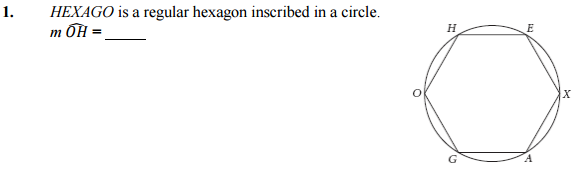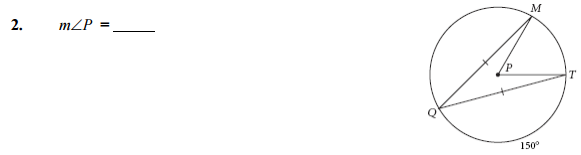# Discovering Chord Properties

## In this lesson you will discover some properties of chords and their central angles. Investigat

1. Drag different points on your figure (B, C, D) to confirm if the chords you constructed stay congruent. Measure central angles CAB and DAE. (Remember, to measure an angle, make sure the vertex is the second point you select.) Write a conjecture about congruent chords in a circle and the central angles they determine (Chord Central Angles Conjecture). 2. The measure of a minor arc is defined as the measure of its central angle. Measure the arc to confirm this. Measure the arc intercepted by the other chord. What can you conclude about congruent chords in a circle and the arcs they intercept? Write a conjecture (Chord Arcs Conjecture).

Chord Central Angles Conjecture: If two chords in a circle are congruent, then they determine two central angles that are ___________.

Check all that apply

Chord Arcs Conjecture: If two chords in a circle are congruent, then their _________________ are congruent.

Check all that applyCheck all that applyCheck all that apply